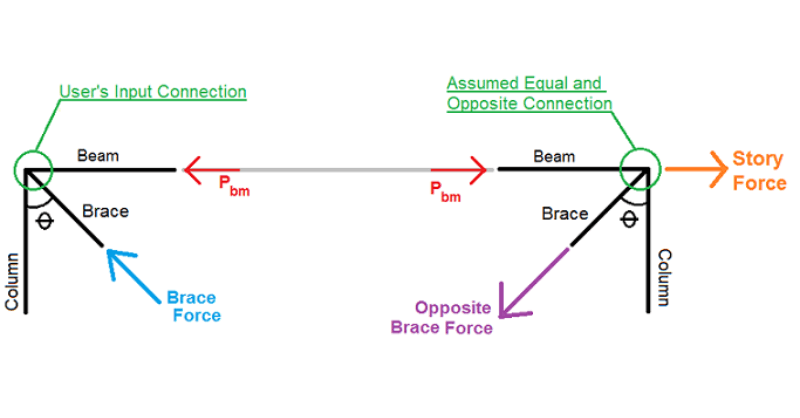# Why Do Seismic Brace Story Forces Change My Existing Code Checks?

It is possible for seismic (OCBF or SCBF) vertical braced connections to have some limit state code checks lower than those for the same non-seismic connection.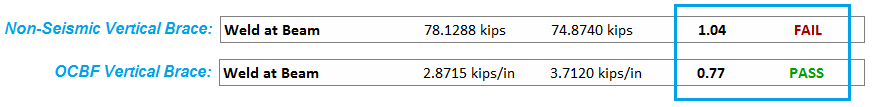This is due to the assumptions that RISAConnection makes when calculating the axial load in the beam. Non-seismic vertical brace connections only have a single beam axial load input value. Because seismic vertical brace connections require separate frame analyses of the various possible load directions, it is not appropriate to use a single input brace axial load for all load direction cases. Doing so could cause un-conservative results.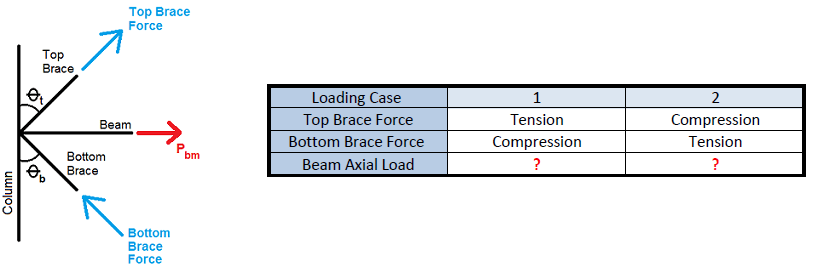#### Story Force and Beam Axial Force Calculation

The beam axial load calculation is done for each applicable loading direction case. To see the details of these calculations, please see the Seismic Load Distribution on the new Seismic tab of the results report.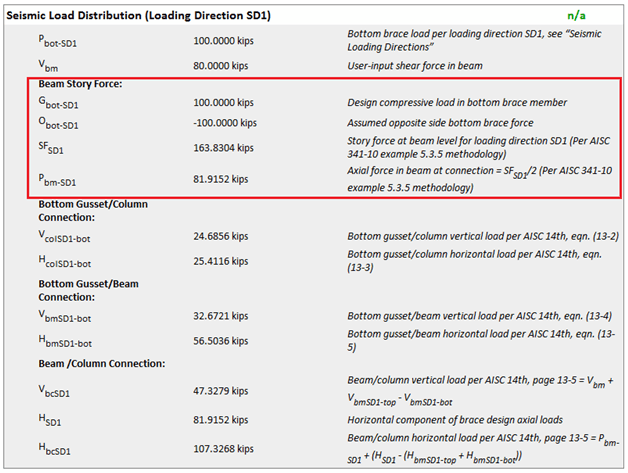The procedure is based on that used in example 5.3.5 of the AISC Seismic Design Manual. It is determined using simple statics and the following assumption:

The input connection is mirrored on the other side of the bay with an equal and opposite braced connection.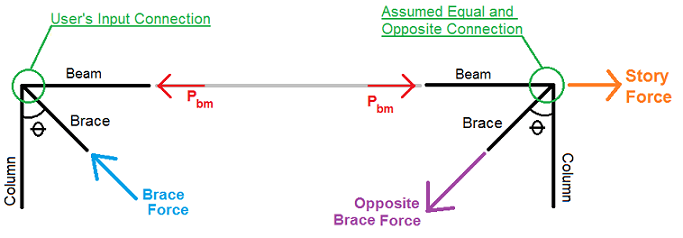Assuming this assumption, the story force and axial force are calculated as: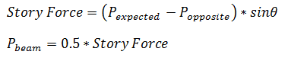#### How is the Beam Axial Force Used?

Once the beam axial load is calculated, it is used to calculate the axial force at the beam to column sub-connection interface. Per the Uniform Force Method, the axial force at the beam to column sub-connection is calculated as: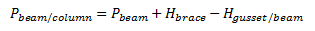• Hbrace = horizontal component of the brace force

• Hgusset/beam = horizontal load at beam to gusset interface per UFM (AISC Steel Design Manual equation 13-5)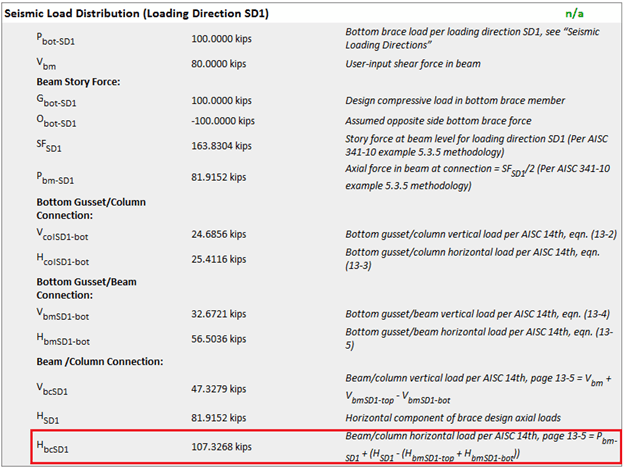This is calculated for each of the applicable loading directions and then the design is done per the governing values; both in tension and compression, for the applicable limit states.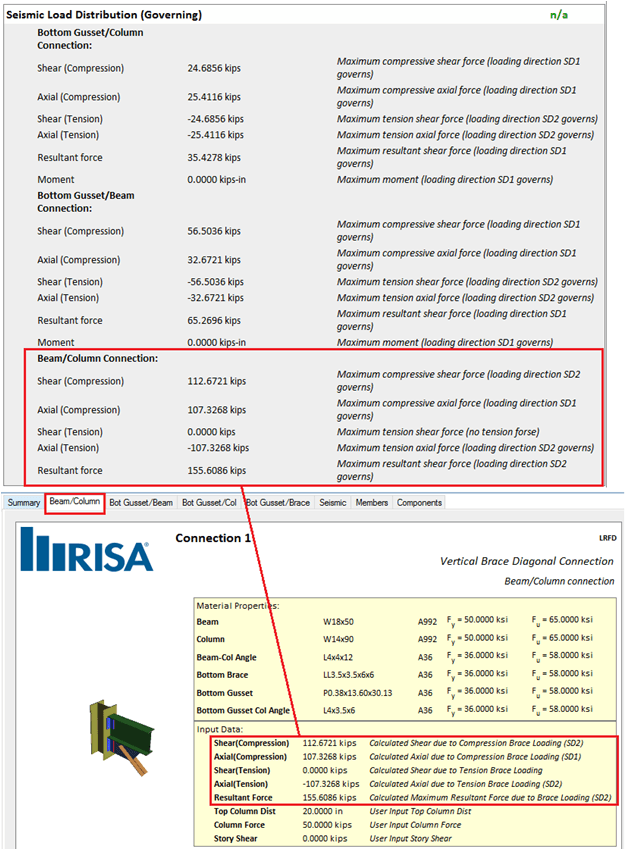This means that the governing axial and/or resultant forces at the sub-connection interfaces can potentially be lower per the seismic analysis than they are for the same non-seismic connection.